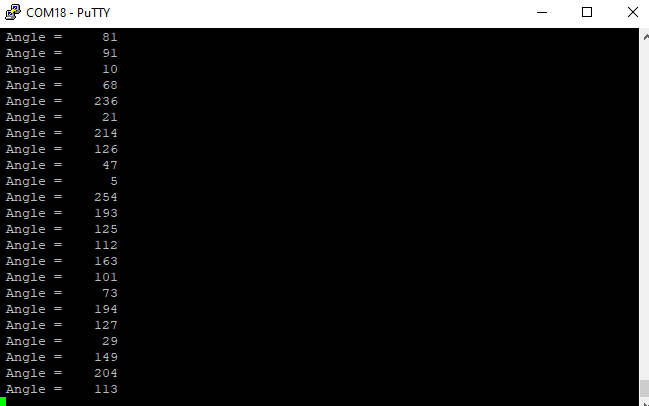# PID control problem

To determine the setpoint of the PID algorithm, I chose to display the data on a USART terminal and see what value it displays when the robot is lying down and what value it displays when the robot stands upright. I have problems displaying this data because when the robot is motionless, data is continuously transmitted. I don’t know if the problem is from the data transmission or I should apply a filter.

In the picture I highlighted this.Hello.

Can you explain what hardware you are using and post the program that is producing the result you shared?

- Patrick

This is my code. The code is made in eclipses and the data is retrieved with the help of usart.

``````/*Acc data transformation function in degrees*/
void AngleAcc (void)
{
Acc_angle_y  = ((atan2(out_z_acc,out_x_acc)) * Transf_Rad_Deg);// angle y aaccelerometer
}

/*Gyro data transformation function in degrees*/
void AngleGyro(void)
{
Gyro_angle_y = out_y_gyro * dt; //angle y gyro. dt = 10 ms sample rate!
}
``````

This is the I2c function for read 2 byte

``````void LSM6DS33_ReadByte( int16_t RegAddress, uint8_t *recv_data)
{

I2C_Start(); // Function to send start condition
I2C_write_data(RegAddress); // Function to write data in slave(SUB)
I2C_RStart();

/*Functie Citire date */
/* Trigger transmit */
TWCR = (1<<TWINT)|(1<<TWEN);
while((TWCR & (1<<TWINT)) == 0);
*recv_data=TWDR;

/* Trigger transmit */
TWCR = (1<<TWINT)|(1<<TWEN);
/* Wait for data to be sent */
while((TWCR & (1<<TWINT)) == 0);

I2C_Stop();

I2C_RStart();

//Moving to the next register
/* Trigger transmit */
TWCR = (1<<TWINT)|(1<<TWEN);
/* Wait for register address to be sent */
while(!(TWCR & (1<<TWINT)));

I2C_RStart();

/* Trigger transmit */
TWCR = (1<<TWINT)|(1<<TWEN);
while((TWCR & (1<<TWINT)) == 0);

/* Load Data to be read and transforms to 16 bits  */
*recv_data|=(((uint16_t)TWDR)<<8);
/* Trigger transmit */
TWCR = (1<<TWINT)|(1<<TWEN);
/* Wait for data to be sent */
while((TWCR & (1<<TWINT)) == 0);

I2C_Stop();

}

/*Function for write */
void LSM6DS33_WriteByte(int16_t RegAddress, int16_t Value )
{
I2C_Start(); // Function to send start condition
I2C_write_data(Value); //Data to write
I2C_Stop(); // Function to send Stop Condition
}

{

}

{
LSM6DS33_R
``````

It sounds like you are probably using a Balboa robot. Is that correct? If so, have you tried running any of the example programs from our Arduino library?

Can you post your complete program, or more ideally, a simplified version of it that demonstrates the problem?

- Patrick

That is the problem. I don’t know where is the error. I want to know the value when the robot is lying down and the value when the robot is standing upright. The first picture shows the angle around the y-axis of the accelerometer. I expect it to be a constant value in both cases. In this case when the robot is lying down I get values from 0 to over 200 and I would expect it to be a single value.

Can you answer the questions for my previous post? We will not be able to offer any troubleshooting advice until we know for sure what hardware you are using.

Also, if you post your complete code, I might be able to offer some suggestions for how to simplify it.

- Patrick